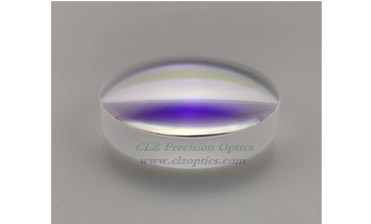# Application of lens in magnifying glass and reading glasses

Nov. 06, 2019

Plano-Concave Lenses is also known as a negative-lens lens. The lens is thin in the middle and thick at the edges. It is also called a concave lens. The concave lens has a diverging effect on the light and can be used as a myopia mirror or an astigmatism mirror.Plano-Concave Lenses

The convex lens is made according to the principle of light refraction. The convex lens is a lens with a thick center and a thinner edge. The convex lens is divided into a double convex shape, a Plano-Convex Lenses and a concave-convex (or a positive meniscus). The convex lens has a converging light effect, so it is also called a converging lens, and a thick convex lens has a telescopic and converging effect, which is related to the lens. Thickness related, you can do magnifying glass, reading glasses.

These features of the lenticular mirror also determine its application in magnifying glasses and reading glasses.

We know that the lenticular lens includes a convex lens and a concave lens. Then the two products, the convex lens, and the concave lens can be seen literally, which are completely different products. So how do you distinguish the two products?

1. Different effects on light

The convex lens mainly concentrates on the light; the concave lens mainly emits light.

2. Different imaging

The convex lens can be an erect magnified virtual image, an inverted magnified real image, an inverted real image, and an inverted real image; the concave lens can only be erected to reduce the virtual image.

3. Different focus

The convex lens has a solid focus and has two focal points; the concave lens has a virtual focus.

4. Different uses

Convex lenses are used for hyperopic glasses; concave lenses are used for myopia glasses.

Now tell everyone a different way to distinguish convex mirrors:

1. Touch method (the middle thin edge is a concave lens, and the middle thick edge is a convex lens)

2. Focus method (injecting parallel light, converging is a convex lens, diverging is a concave lens)

3. Magnification method (put the lens on the word, the word after the photo is enlarged is a convex lens, and the reduction is a concave lens)

4. Shake method (put the lens on the word and move to one side, the direction of the word is the same as the lens moving direction is the concave lens, the opposite is the convex lens)

The concave lens is also called a negative spherical lens. The center of the lens is thin and the periphery is thick and concave. Therefore, it is also called a concave lens. The concave lens has a diverging effect on the light. After the parallel rays are deflected by the concave spherical lens, the light diverges and becomes divergent rays. It is impossible to form a solid focus. The reverse extension line along the diffused light passes through the F point on the same side of the projected light. A virtual focus (the concave lens has two virtual focus).

Our company is Dome lens Manufacturer, welcome to come to us.